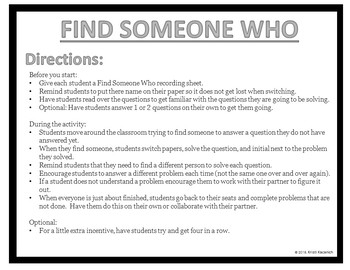# Find Someone Who - 4th Grade End of Year ReviewSubject
Resource Type
File Type
PDF (682 KB|4 pages)
Standards
\$2.00
• Product Description
• Standards
Find Someone Who is a great student engagement activity that get students out of their seats, collaborating with other students, and practicing math.

This end of year review covers multiple 4th grade Common Core standards over a series of 16 questions. This product also include a direction page, a student work space, and a teacher answer key.
Compare two decimals to hundredths by reasoning about their size. Recognize that comparisons are valid only when the two decimals refer to the same whole. Record the results of comparisons with the symbols >, =, or <, and justify the conclusions, e.g., by using a visual model.
Express a fraction with denominator 10 as an equivalent fraction with denominator 100, and use this technique to add two fractions with respective denominators 10 and 100. For example, express 3/10 as 30/100, and add 3/10 + 4/100 = 34/100.
Apply and extend previous understandings of multiplication to multiply a fraction by a whole number.
Add and subtract mixed numbers with like denominators, e.g., by replacing each mixed number with an equivalent fraction, and/or by using properties of operations and the relationship between addition and subtraction.
Compare two fractions with different numerators and different denominators, e.g., by creating common denominators or numerators, or by comparing to a benchmark fraction such as 1/2. Recognize that comparisons are valid only when the two fractions refer to the same whole. Record the results of comparisons with symbols >, =, or <, and justify the conclusions, e.g., by using a visual fraction model.
Total Pages
4 pages